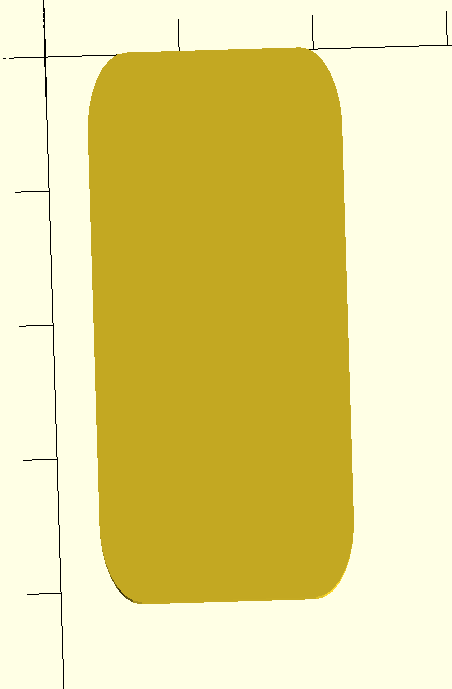Skip to content

Instantly share code, notes, and snippets.

#groovenectar/roundedcube.scad Last active Jul 20, 2019

roundedcube.scad - Fork me and make me better!
 // More information: https://danielupshaw.com/openscad-rounded-corners/ // Set to 0.01 for higher definition curves (renders slower) \$fs = 0.15; module roundedcube(size = [1, 1, 1], center = false, radius = 0.5, apply_to = "all") { // If single value, convert to [x, y, z] vector size = (size == undef) ? [size, size, size] : size; translate_min = radius; translate_xmax = size - radius; translate_ymax = size - radius; translate_zmax = size - radius; diameter = radius * 2; obj_translate = (center == false) ? [0, 0, 0] : [ -(size / 2), -(size / 2), -(size / 2) ]; translate(v = obj_translate) { hull() { for (translate_x = [translate_min, translate_xmax]) { x_at = (translate_x == translate_min) ? "min" : "max"; for (translate_y = [translate_min, translate_ymax]) { y_at = (translate_y == translate_min) ? "min" : "max"; for (translate_z = [translate_min, translate_zmax]) { z_at = (translate_z == translate_min) ? "min" : "max"; translate(v = [translate_x, translate_y, translate_z]) if ( (apply_to == "all") || (apply_to == "xmin" && x_at == "min") || (apply_to == "xmax" && x_at == "max") || (apply_to == "ymin" && y_at == "min") || (apply_to == "ymax" && y_at == "max") || (apply_to == "zmin" && z_at == "min") || (apply_to == "zmax" && z_at == "max") ) { sphere(r = radius); } else { rotate = (apply_to == "xmin" || apply_to == "xmax" || apply_to == "x") ? [0, 90, 0] : ( (apply_to == "ymin" || apply_to == "ymax" || apply_to == "y") ? [90, 90, 0] : [0, 0, 0] ); rotate(a = rotate) cylinder(h = diameter, r = radius, center = true); } } } } } } }
Owner Author

### groovenectar commented Jul 23, 2015

 Usage: ``````// A single integer creates a cube with the specified wall distance. Default [1, 1, 1] size = 5; // An [x, y, z] vector specifies distance on each axis. Default [1, 1, 1] size = [2, 3, 5]; // Whether or not to place the object centered on the origin. Default false center = true|false; // Specify a rounding radius. Default 0.5 radius = 0.5 // Specify where to apply the rounded corners. Default "all" apply_to = "all"|"x"|"y"|"z"|"zmax"|"zmin"|"xmax"|"xmin"|"ymax"|"ymin" roundedcube(size, center, radius, apply_to); `````` Examples: ``````color("Yellow") roundedcube(3, true, 0.7, "xmin"); translate(v = [1, 0, 2]) color("Pink") roundedcube([4, 2, 2], false, 0.6, "zmax"); translate(v = [-4, -1, 2]) color("Lightblue") roundedcube(2, false); translate(v = [0, 0, 6]) color("Orange") roundedcube([3, 2, 2], true, 0.2); translate(v = [2.5, -0.5, 5]) color("Green") roundedcube([3, 2, 2], false, 0.4, "z"); `````` Output:### thunfischbrot commented Dec 20, 2015

 Hi Daniel, thank you for your work! If I am not mistaken there is a bug which doesn't let you create a shape with rounded corners which extends into any direction for less than twice the radius of the rounded corners. The bug is that the x|y|z >= radius*2 should only apply to the dimensions which you apply the rounded corners to. Or am I mistaken? Demo: ``````use translate([0,-30,0]) color("Green") roundedcube([40,10,1], true, .1, "z"); translate([0,-15,0]) color("Orange") roundedcube([40,10,1], true, .5, "z"); color("Blue") roundedcube([40,10,1], true, 1, "z"); translate([0,15,0]) color("Red") roundedcube([40,10,1], true, 4, "z"); ``````### rbuckland commented Jan 8, 2017 • edited

 Brilliant !thank you. I have been meaning to write an uber version (optional rounded faces etc). You nailed it :-)

### Diaoul commented Feb 3, 2018

 Forked to add scaling for when you don't want the radius to be the same for x and y for example. ``````roundedcube([41, 22, 23], radius=6, scale_y=1/2, apply_to="z"); ``````### rfinz commented Jan 18, 2019

 excellent little script! consider this a +1! 👍
to join this conversation on GitHub. Already have an account? Sign in to comment
You can’t perform that action at this time.